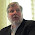## Sunday, July 12, 2015

### Dimensional Analysis a Simple but Effective Tool

Dimensional Analysis (DA), sometimes called unit analysis, is a method by which modelers or other technologists keep track of the units of numbers during mathematical operations.   It is a reality check in that it tells you if the values(s) that you use and calculate make any sense or not.   Indeed, it has saved me many times from making embarrassing mistakes.  Granted, I still make embarrassing mistakes but it happens less often because of DA.  Also, I have gotten smart enough to always require peer review and sign-off for important work.

Conceptually DA is relatively easy to understand.  You simply need to  know what units are associated with the quantities you are working with and then do the algebra to cancel them out.

Of course, knowing the units is first. For example, length is always expressed in some common measure like: inch, foot, yard, meter etc.    Area has units of length squared like square meters or m2.   Volume is length cubed; for example, m3.

You do the same for mass:  e.g., grams (g), pounds (lbs).

The quantity “time” is the most interesting and, by definition, most dynamic: e.g.,  seconds (s), minutes (min), hours (hrs), years (yr).

The first step in DA is understanding the conversion factors for the various units within each quantity.  For example: 0.001 mg = 1 microgram (ug) = 1,000 nanograms (ng) = 1,000,000 picograms (pg).

Ultimately, the quantities get combined into the common entities that we know and love: e .g.,  Speed = miles/hr,  Concentration =  g/m3.

The real fun comes when they get combined into more complicated algorithms as is often done in modeling.   For example,  image trying to understand and sort out the following modeling algorithm which describes the dynamic point-in-time concentration in an air volume in a well-mixed box containing a spill:

Here we need to sort things out by doing DA

You would need to know the units of α, M0, V, Q and t.  Which are

M0 = initial spill mass (mg)
α = evaporation rate constant (1/min)
V = effective room volume (m3)
Q = ventilation rate (m3/min)
t = time (min)

Let's start but taking "bite sized" pieces of the equation:

The quantity (α) (M0) has the units of (1/min)(mg) or, when combined they render mg/min.   The quantity (α)(V) – Q has the units of (1/min)(m3) or, when combined, m3/min.    If you divide these units into into the units for (α) (M0) you get mg/min divided by m3/min which cancels out the minutes (min) to give mg/m3 which happens to be the units of C.

The units in the exponents factor completely cancel out so they are “unitless”.   Thus "e" to a unitless power is a unitless number as well.

Two excellent tutorials on DA online are:

The first part of the second url is somewhat animated and I found it to be particularly clear.  You may want to skip the part on the use of DA for chemical reactions but  it is quite interesting.

If you have avoided diving into this sort of analysis in the past, I urge you to consider these tutorials (or others you might find online), once you understand these concepts you will be able to analyze any algorithm for its units to see if it, its numbers and its predictions make sense.

I love to get comments back from the readers of this blog.  I would really like to know if this subject was of any value or perhaps aimed too low.  I simply know that DA has been a excellent tool for me over the years and wanted to share it.

1.Great post! I can't count the number of errors I've found in PBPK models that could have been avoided by a DA check. I now do it routinely when performing verification of any biological model.

2.Thanks Conrad, PBPK is hard enough with the correct units!

3.Sorry to be anonymous, but I don't have an on-line identity I can use for this, as far as I know.

Back in high school in 1966, my physics teacher, a former General Electric engineer from their jet engine operations, taught us Dimensional Analysis, but didn't give the process a name. Nevertheless I've been using it almost automatically since then, through Astonomy and into Ventilation and Industrial Hygiene.

I have always been mystified as to why it seems to be a secret, or at least not well known.